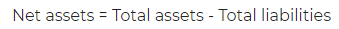# Net Assets – Definition, Formula and Example

Net assets is the difference of total assets and total liabilities of a firm.

#### Net Assets Formula:#### Net Assets Example:

The accountant of XYX Ltd comes with the below figure.

Total assets of company = INR 10,00,000

Total liabilities of company = INR 7,00,000

Management asks to calculate the net assets. He follows the order and makes his calculation as given below:

Net Assets = 10,00,000 – 7,00,000 = INR 3,00,000

Posted on Categories Dictionary